# DAV Class 4 Maths Chapter 6 Brain Teasers Solutions

The DAV Class 4 Maths Book Solutions and DAV Class 4 Maths Chapter 7 Brain Teasers Solutions of Weight offer comprehensive answers to textbook questions.

## DAV Class 4 Maths Ch 6 Brain Teasers Solutions

Question 1.
Convert the following into grams.
(i) 10 kg 7 g
10 kg 7 g
= 10 × 1000 g + 7 g (1kg = 1000g)
= 10,000 g + 7 g
= 10007 g

(ii) 105 kg 75 g
105 kg 75 g
= 105 × 1000 g + 75 gm
= 105000 g + 75 g
= 105075 g

(iii) 6 kg 52 g
6 kg 52 g
= 6 ×1000 + 52 g
= 6000 g + 52 g
= 6052 g.Question 2.
Convert the following into kilograms and grams.
(a) 12075 g
12075 g
= 12 kg 75 gm

(b) 57613 g
57613 g
= 57 kg 613 g

(c) 120765 g
120765 g
= 120 kg 765 g

(d) 250330 g
250330 g
= 250 kg 330 g

Question 3.
(a) 51 kg 520 g and 49 kg 630 g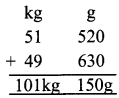101 kg 150 g

(b) 31 kg 635 g and 140 kg 170 g171 kg 805 g

(c) 54 kg 320 g and 79 kg 520 g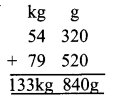133 kg 840 g

Question 4.
Find the difference:
(a) 76 kg 540 g and 89 kg 740 g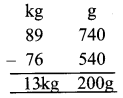13 kg 200 g

(b) 8 kg 70 g and 12 kg 703 g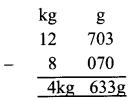4 kg 633 g

(c) 120 kg 270 g and 160 kg 320 g40 kg 50 gQuestion 5.
Ramil bought 75 kg 846 g of wheat from the wholeseller. He also bought 16 kg 790 g soya from wholeseller. Find the total weight of wheat and soya bought by him.
Quantity of wheat bought = 75kg 846
Quantity of soya bought = 16 790
Total weight = 92kg 636g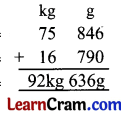92 kg 636 g.

Question 6.
A fruit seller has 75 kg 840 g apples in morning. 46 kg 658 g apples are sold till after¬noon. What quantity of apples left in evening.